Courses

# Legendre Special Function (Part - 4) - Mathematical Methods of Physics, UGC - NET Physics Physics Notes | EduRev

## Physics for IIT JAM, UGC - NET, CSIR NET

Created by: Akhilesh Thakur

## Physics : Legendre Special Function (Part - 4) - Mathematical Methods of Physics, UGC - NET Physics Physics Notes | EduRev

The document Legendre Special Function (Part - 4) - Mathematical Methods of Physics, UGC - NET Physics Physics Notes | EduRev is a part of the Physics Course Physics for IIT JAM, UGC - NET, CSIR NET.
All you need of Physics at this link: Physics

ASSOCIATED LEGENDRE FUNCTIONS

When Helmholtz’s equation is separated in spherical polar coordinates, one of the separated ODEs is the associated Legendre equation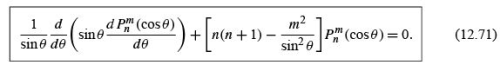With x = cos θ , this becomes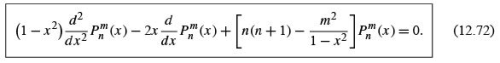If the azimuthal separation constant m= 0, we have Legendre’s equation, Eq. (12.28).
The regular solutions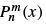(with m not necessarily zero, but an integer) are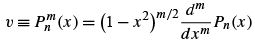(12.73a)

with m ≥ 0 an integer

One way of developing the solution of the associated Legendre equation is to start with the regular Legendre equation and convert it into the associated Legendre equation by using multiple differentiation. These multiple differentiations are suggested by Eq. (12.73a).
We take Legendre’s equation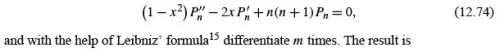(1 − x 2)u′′ − 2x(m + 1)u′ + (n − m)(n + m + 1)u = 0,       (12.75)

where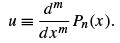(12.76)

Equation (12.74) is not self-adjoint. To put it into self-adjoint form and convert the weighting function to 1, we replace u(x ) by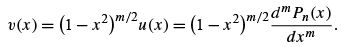(12.73b)

Solving for u and differentiating, we obtain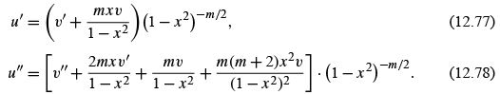Substituting into Eq. (12.74), we ﬁnd that the new function v satisﬁes the self-adjoint ODE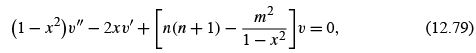which is the associated Legendre equation; it reduces to Legendre’s equation when m is set equal to zero. Expressed in spherical polar coordinates, the associated Legendre equation is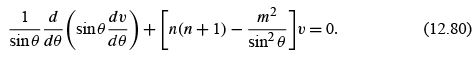Associated Legendre Polynomials

The regular solutions, relabeled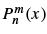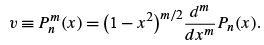(12.73c)

These are the associated Legendre functions. Since the highest power of x in Pn (x ) is x n ,wemusthave m ≤ n (or the m-fold differentiation will drive our function to zero).
In quantum mechanics the requirement that m ≤ n has the physical interpretation that the expectation value of the square of the z component of the angular momentum is less than or equal to the expectation value of the square of the angular momentum vector L,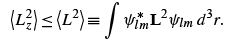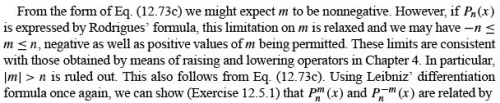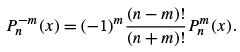From our deﬁnition of the associated Legendre functions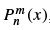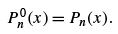(12.82)

A generating function for the associated Legendre functions is obtained, via Eq. (12.71), from that of the ordinary Legendre polynomials: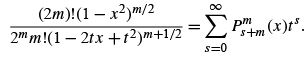(12.83)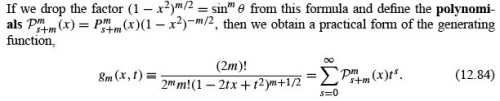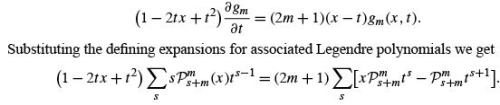Comparing coefﬁcients of powers of t in these power series, we obtain the recurrence relation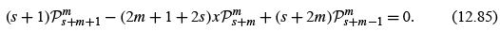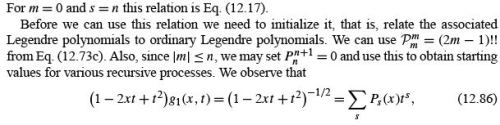so upon inserting Eq. (12.84) we get the recursion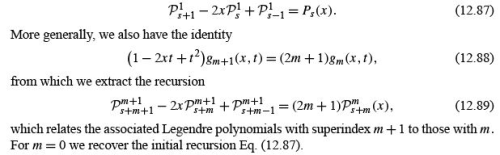Table 12.2 Associated Legendre Functions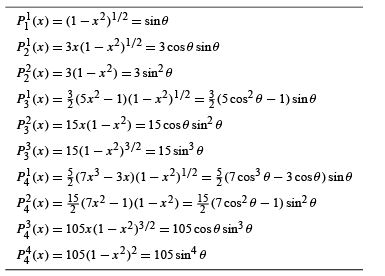Example 12.5.1 LOWEST ASSOCIATED LEGENDRE POLYNOMIALS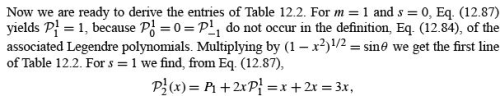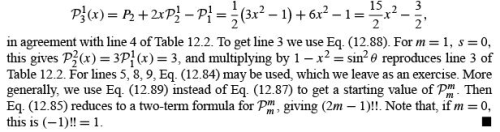Example 12.5.2 SPECIAL VALUES

For x = 1we use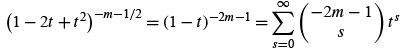in Eq. (12.84) and ﬁnd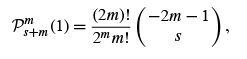(12.90)

where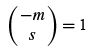for s = 0 and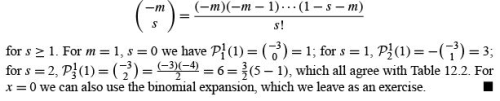Recurrence Relations

As expected and already seen, the associated Legendre functions satisfy recurrence relations. Because of the existence of two indices instead of just one, we have a wide variety of recurrence relations: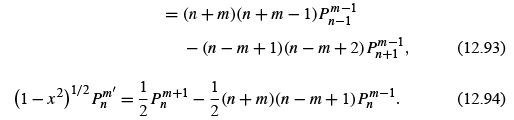These relations, and many other similar ones, may be veriﬁed by use of the generating function (Eq. (12.4)), by substitution of the series solution of the associated Legendre equation (12.79) or reduction to the Legendre polynomial recurrence relations, using Eq. (12.73c). As an example of the last method, consider Eq. (12.93). It is similar to Eq. (12.23):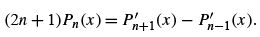(12.95)

Let us differentiate this Legendre polynomial recurrence relation m times to obtain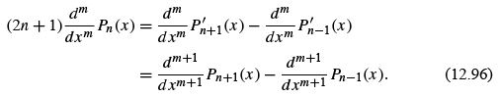Now multiplying by (1 − x 2 )(m+1)/2 and using the deﬁnition of Pn (x ), we obtain the ﬁrst part of Eq. (12.93).

Parity

The parity relation satisﬁed by the associated Legendre functions may be determined by examination of the deﬁning equation (12.73c). As x →−x , we already know that Pn (x ) contributes a (−1)n .The m-fold differentiation yields a factor of (−1)m . Hence we have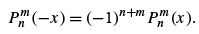(12.97)

A glance at Table 12.2 veriﬁes this for 1 ≤ m ≤ n ≤ 4.
Also, from the deﬁnition in Eq. (12.73c),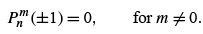(12.98)

Orthogonality

The orthogonality of the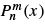follows from the ODE, just as for the Pn (x ) (Section 12.3), if m is the same for both functions. However, it is instructive to demonstrate the orthogonality by another method, a method that will also provide the normalization constant.
Using the deﬁnition in Eq. (12.73c) and Rodrigues’ formula (Eq. (12.65)) for Pn (x ),we ﬁnd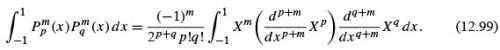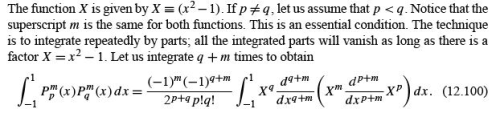The integrand on the right-hand side is now expanded by Leibniz’ formula to give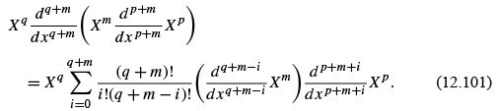Since the term Xcontains no power of x greater than x2m ,wemusthave

q + m − i ≤ 2m                    (12.102)

or the derivative will vanish. Similarly,

p + m + i ≤ 2p.               (12.103)

q ≤ p,                                 (12.104)

which contradicts our assumption that p< q . Hence, there is no solution for i and the integral vanishes. The same result obviously will follow if p> q .
For the remaining case, p = q , we have the single term corresponding to i = q − m.
Putting Eq. (12.101) into Eq. (12.100), we have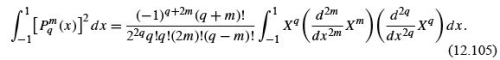Since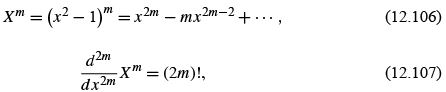Eq. (12.105) reduces to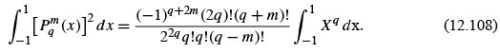The integral on the right is just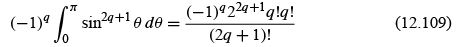(compare Exercise 8.4.9). Combining Eqs. (12.108) and (12.109), we have the orthogonality integral,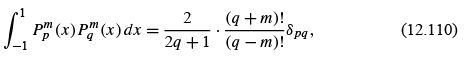or, in spherical polar coordinates,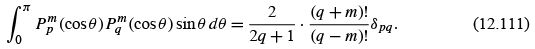The orthogonality of the Legendre polynomials is a special case of this result, obtained by setting m equal to zero; that is, for m = 0, Eq. (12.110) reduces to Eqs. (12.47) and (12.48). In both Eqs. (12.110) and (12.111), our Sturm–Liouville theory of Chapter 10 could provide the Kronecker delta. A special calculation, such as the analysis here, is required for the normalization constant.
The orthogonality of the associated Legendre functions over the same interval and with the same weighting factor as the Legendre polynomials does not contradict the uniqueness of the Gram–Schmidt construction of the Legendre polynomials.
Table 12.2 suggests that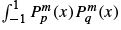dx may be written as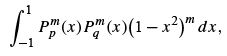where we deﬁned earlier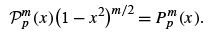The functions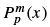may be constructed by the Gram–Schmidt procedure with the weighting function w(x ) = (1 − x 2 )m .
It is possible to develop an orthogonality relation for associated Legendre functions of the same lower index but different upper index. We ﬁnd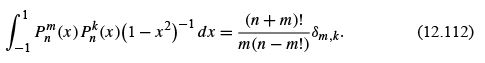Note that a new weighting factor, (1 − x 2 )−1 , has been introduced. This relation is a mathematical curiosity. In physical problems with spherical symmetry solutions of Eqs. (12.80) and (9.64) appear in conjunction with those of Eq. (9.61), and orthogonality of the azimuthal dependence makes the two upper indices equal and always leads to Eq. (12.111).

Offer running on EduRev: Apply code STAYHOME200 to get INR 200 off on our premium plan EduRev Infinity!

159 docs

,

,

,

,

,

,

,

,

,

,

,

,

,

,

,

,

,

,

,

,

,

,

,

,

;